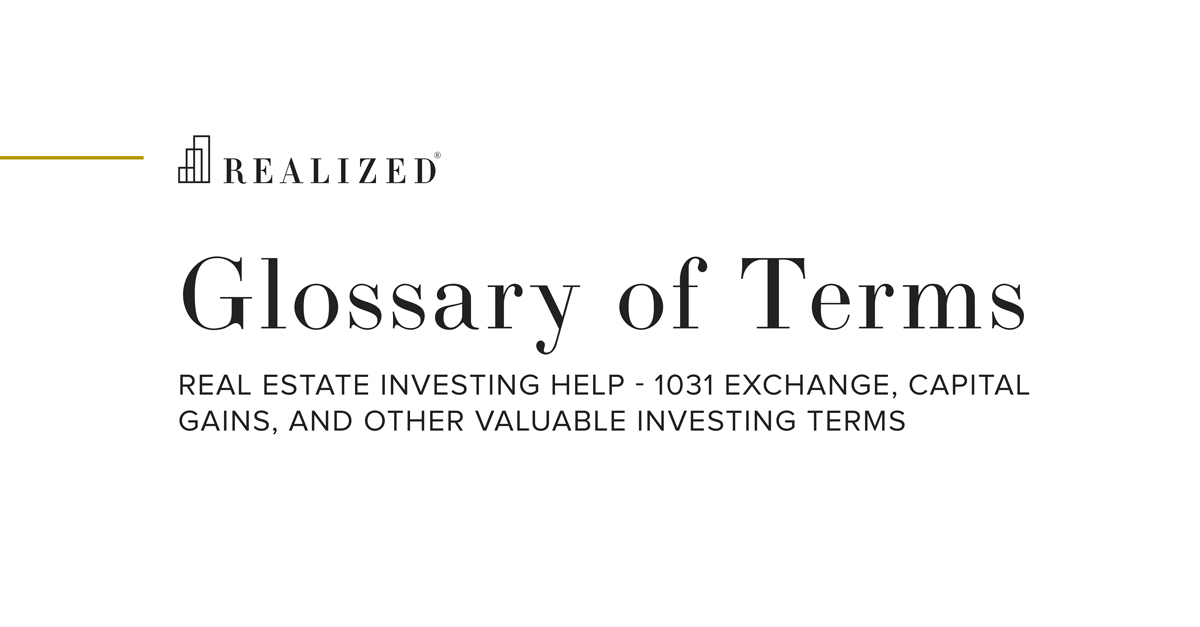Rate Of Return 2019-06-03 08:00:00

Rate of return is the profit or loss on an investment over a specified period of time expressed as proportion of the investment amount. A rate of return may be depicted for one period only, such as an annual rate of return, or may include total cash flows received as well as an increase or decrease in value.

As an example calculation, an investment purchased for \$100 which generates cash flow of \$5 for one year and is then sold for \$110 would generate an annual rate of return of 5.0% (\$5 of cash flow divided by \$100 investment) and a total rate of return of 15.0% (\$5 in cash flow plus \$10 in appreciation equals \$15 divided by \$100 equals 15%). Also known as yield.

### The Investor's Guidebook To Net Lease